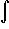#Interactive Real Analysis

Next | Previous | Glossary | Map

## 7.4. Lebesgue Integral

### Example 7.4.4(a): Lebesgue Integral for Simple Functions

Find the Lebesgue integral of the constant function f(x) = c over the interval [a, b].
The constant function f(x) = c can be written as a simple function
f(x) = c XR(x)
Therefore, the (Lebesgue) integral of f over [a, b] is by definition:[a, b] f(x) dx =X[a, b](x) f(x) dx =
=c X[a, b](x) dx = c m([a, b]) = c (b - a)
which is the same answer as for the Riemann integral.
Next | Previous | Glossary | Map